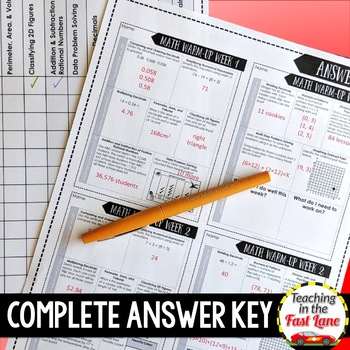# Weekly Math Review 5th Grade FREE One Week SampleSubject
Resource Type
File Type
PDF (1 MB|10 pages)
Standards
FREE
• Product Description
• Standards
This math review resource is a 36 week long daily review of the important math concepts for 5th grade. I designed it based on the twelve readiness standards outlined in the TEKS then aligned it to CCSS.

Check out the complete resource here.

This resource includes:
•Teacher Directions
•Tips for Implementation
•A Layout Key
•CCSS Alignment Key
•TEKS Alignment Key
•I Can Statements
•36 Weeks of Weekly Review
•Student Data Tracking Pages

This resource is ready to print and go!

Why Do I Need This Resource?
Practice Makes Permanent!
Each day students will review 2-3 math concepts to keep their learning fresh and preview standards before you get to them in the year. The format each week stays the same, but the problems change to continue to challenge students all year long!

Topics Covered in this Review Each Week-
★Mondays

→Comparing and Ordering Decimals
→Simplifying Numerical Expressions
★Tuesdays
→Multiplying Decimals
→Perimeter, Area, and Volume
→Classifying 2D Figures
★Wednesdays
→Addition and Subtraction of Positive Rational Numbers
→Data Problem Solving
★Thursdays
→Dividing Decimals
→Numerical Patterns
→Division with Unit Fractions
★Fridays
→Multi-Step Problem Solving
→Graphing Ordered Pairs
Classify two-dimensional figures in a hierarchy based on properties.
Use a pair of perpendicular number lines, called axes, to define a coordinate system, with the intersection of the lines (the origin) arranged to coincide with the 0 on each line and a given point in the plane located by using an ordered pair of numbers, called its coordinates. Understand that the first number indicates how far to travel from the origin in the direction of one axis, and the second number indicates how far to travel in the direction of the second axis, with the convention that the names of the two axes and the coordinates correspond (e.g., 𝘹-axis and 𝘹-coordinate, 𝘺-axis and 𝘺-coordinate).
Interpret division of a whole number by a unit fraction, and compute such quotients. For example, create a story context for 4 ÷ (1/5), and use a visual fraction model to show the quotient. Use the relationship between multiplication and division to explain that 4 ÷ (1/5) = 20 because 20 × (1/5) = 4.
Interpret division of a unit fraction by a non-zero whole number, and compute such quotients. For example, create a story context for (1/3) ÷ 4, and use a visual fraction model to show the quotient. Use the relationship between multiplication and division to explain that (1/3) ÷ 4 = 1/12 because (1/12) × 4 = 1/3.
Find the area of a rectangle with fractional side lengths by tiling it with unit squares of the appropriate unit fraction side lengths, and show that the area is the same as would be found by multiplying the side lengths. Multiply fractional side lengths to find areas of rectangles, and represent fraction products as rectangular areas.
Total Pages
10 pages